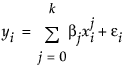Publication date: 08/13/2020

## Fit Line

The Fit Line option finds the parameters β0 and β1 for the straight line that fits the points to minimize the residual sum of squares. The model for the ith row is written yi = β0 + β1xi + εi.

A polynomial of degree 2 is a parabola; a polynomial of degree 3 is a cubic curve. For degree k, the model for the ith observation is as follows: